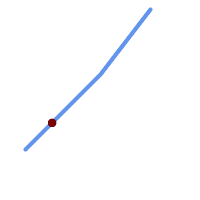## Name

ST_LineInterpolatePoint — Returns a point interpolated along a line at a fractional location.

## Synopsis

`geometry ST_LineInterpolatePoint(`geometry a_linestring, float8 a_fraction`)`;

## Description

Returns a point interpolated along a line at a fractional location. First argument must be a LINESTRING. Second argument is a float between 0 and 1 representing the fraction of line length where the point is to be located. The Z and M values are interpolated if present.

See ST_LineLocatePoint for computing the line location nearest to a Point.This function computes points in 2D and then interpolates values for Z and M, while ST_3DLineInterpolatePoint computes points in 3D and only interpolates the M value.Since release 1.1.1 this function also interpolates M and Z values (when present), while prior releases set them to 0.0.

Availability: 0.8.2, Z and M supported added in 1.1.1

Changed: 2.1.0. Up to 2.0.x this was called ST_Line_Interpolate_Point.This function supports 3d and will not drop the z-index.

## ExamplesA LineString with the interpolated point at 20% position (0.20)

```-- The point 20% along a line

SELECT ST_AsEWKT(
ST_LineInterpolatePoint(
'LINESTRING(25 50, 100 125, 150 190)',
0.2 ));

st_asewkt
----------------
POINT(51.5974135047432 76.5974135047432)
```

The mid-point of a 3D line:

```SELECT ST_AsEWKT(
ST_LineInterpolatePoint('
LINESTRING(1 2 3, 4 5 6, 6 7 8)',
0.5 ));

st_asewkt
--------------------
POINT(3.5 4.5 5.5)
```

The closest point on a line to a point:

```SELECT ST_AsText(
ST_LineInterpolatePoint( line.geom,
ST_LineLocatePoint( line.geom, 'POINT(4 3)')))
FROM (SELECT ST_GeomFromText('LINESTRING(1 2, 4 5, 6 7)') As geom) AS line;

st_astext
------------
POINT(3 4)
```